## Friction Questions and Answers Part-2

1. To avoid slipping while walking on ice, one should take smaller steps because of the
a) Friction of ice is large
b) Larger normal reaction
c) Friction of ice is small
d) Smaller normal reaction

Explanation: Friction of ice is small

2. A box is lying on an inclined plane what is the coefficient of static friction if the box starts sliding when an angle of inclination is $60^{\circ}$
a) 1.173
b) 1.732
c) 2.732
d) 1.677

Explanation:3. A block of mass 2 kg is kept on the floor. The coefficient of static friction is 0.4. If a force F of 2.5 Newtons is applied on the block as shown in the figure, the frictional force between the block and the floor will be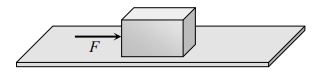a) 2.5 N
b) 5 N
c) 7.84 N
d) 10 N

Explanation: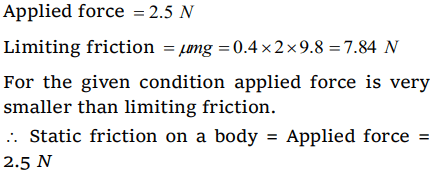4. Which one of the following is not used to reduce friction
a) Oil
b) Ball bearings
c) Sand
d) Graphite

Explanation: Sand is used to increase the friction

5.If a ladder weighing 250N is placed against a smooth vertical wall having coefficient of friction between it and floor is 0.3, then what is the maximum force of friction available at the point of contact between the ladder and the floor
a) 75 N
b) 50 N
c) 35 N
d) 25 N

Explanation: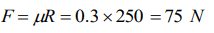6. A body of mass 2 kg is kept by pressing to a vertical wall by a force of 100 N. The coefficient of friction between wall and body is 0.3. Then the frictional force is equal to
a) 6 N
b) 20 N
c) 600 N
d) 700 N

Explanation: For the given condition, Static friction
= Applied force = Weight of body
= 2 * 10 = 20 N

7. A horizontal force of 10 N is necessary to just hold a block stationary against a wall. The coefficient of friction between the block and the wall is 0.2. the weight of the block isa) 2 N
b) 20 N
c) 50 N
d) 100 N

Explanation:8. If mass of A = 10 kg , coefficient of static friction = 0.2, coefficient of kinetic friction = 0.2. Then mass of B to start motion is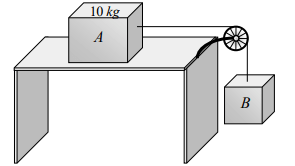a) 2 kg
b) 2.2 kg
c) 4.8 kg
d) 200 gm

Explanation: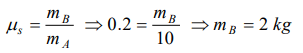9. A uniform metal chain is placed on a rough table such that one end of chain hangs down over the edge of the table. When onethird of its length hangs over the edge, the chain starts sliding. Then, the coefficient of static friction is
a) $\frac{3}{4}$
b) $\frac{1}{4}$
c) $\frac{2}{3}$
d) $\frac{1}{2}$10. A lift is moving downwards with an acceleration equal to acceleration due to gravity. A body of mass m kept on the floor of the lift is pulled horizontally. If the coefficient of friction is $\mu$, then the frictional resistance offered by the body is
b) $\mu mg$
c) 2$\mu mg$